# Python print() Function

The print() function or statement in Python is I think the most used statement in my perspective. Because every time we need to produce or print some output, the print() function is required. This article teaches you everything about this function.

### Definition of print() Function

The print() function is used to produce output. For example:

`print("Hey!\nWelcome to codescracker.com")`

The above program produces exactly same output as shown in the snapshot given below: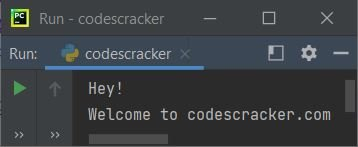## Syntax of print() Function

The syntax to use print() function is:

`print(value(s), sep=' ', end='\n', file=sys.stdout, flush=False)`

Note: Except value(s), all other parameters are optional. Even value(s) is also optional. That is, print() doesn't produce any error if you doesn't provide any parameter to it. But if you doesn't provide any value/expression, then the output will be nothing or blank for that print() statement.

Note: Normally we don't need the last two parameters. The explanation of these two parameters are also given here.

## All Parameters of print() Function

In above syntax of print(). Here are the description of all the parameters one by one.

#### The value(s)/expression(s) Parameter

This is the most used parameter. Any number of value/expression can be passed. That is, the expression passed in print() is not limited to only one. That is, you can pass any number of expressions to print() where expression must be separated by a comma as shown in the program given below:

`print("Hey!", "\nWelcome to codescracker.com")`

produces the same output as of very first program of this article.

Note: The print() function converts all the expressions passed in it, into a string before writing or printing the things to the output screen.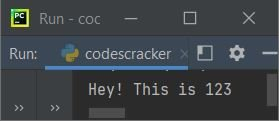#### The sep= Parameter

Now the second parameter is sep=' '. It is used to separate expression using manually assigned character or combination of characters. To learn more about it, refer to sep parameter in Python. If you doesn't use or provide this parameter to print(). Then by default, all expression gets separated by a single space.

#### The end= Parameter

The third parameter is end='\n'. It is used to skip insertion of an automatic newline using print() on the output screen. To learn more about it, refer to end parameter in Python. If you doesn't use it, then after each print() statement, a newline automatically gets inserted on output screen.

#### The file= Parameter

The fourth parameter is file=sys.stdout. This parameter is used to write some content to a file instead of writing on output screen. If you doesn't use this parameter, then the sys.stdout automatically gets used, that prints objects or expressions, on the output screen. Here is an example program that uses file= parameter:

```with open('codescracker.txt', mode='w') as file_object:
print('Hey, Welcome', file=file_object)```

creates a file codescracker.txt inside the current directory and writes Hey, Welcome string to that file. If you open the folder, where the source code of above program is saved. Then you see a file codescracker.txt is available. And if you open that file, it contains Hey, Welcome as content. That is, if you need to write all the expression of print() to a file instead of writing to output screen, you need to use file parameter. Here is the snapshot of the folder where the file is created, along with opened file codescracker.txt: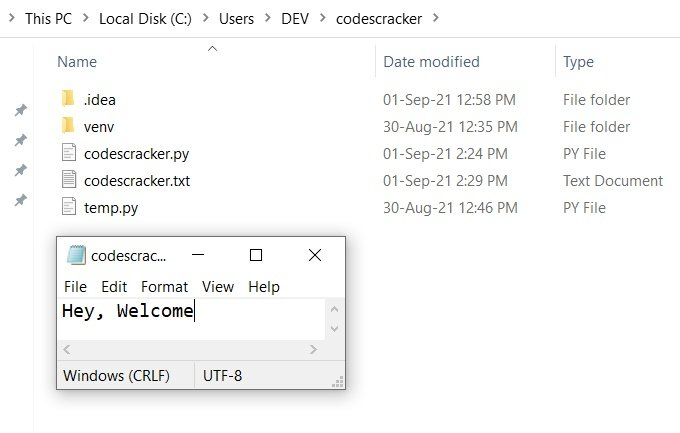Note: To learn about file handling in Python, refer to the separate tutorial on it.

#### The flush= Parameter

The last or the fifth parameter is flush. It is used to flush the stream. The default value of this parameter is False. Specifying True to it, means the output gets written as a sequence of characters one by one. Writing one character at a time is a slow process. Therefore we do not need it until a special requirement occur where we need to write one character at a time.

## print() Function Example

Here is an example of print() function that uses all the parameters as shown in its syntax given above:

```import sys
print("Hello", 123, "How are you?", sep="\n", end="\n", file=sys.stdout, flush=False)```

This program produces exactly the same output as shown in the snapshot given below:Note: Except sep=, all parameter is assigned with its default values in above program.

I've shown you the complete output screen produced by above program. The IDE used is PyCharm. Therefore, if you run the above program in PyCharm, then exactly same output you'll see.

The reason behind showing the complete output is, to mainly show you the use of sep and end. As you can see, each expression say Hello, 123 and How are you? gets separated by a newline using the sep, because sep works on expression for the current print() function where it is available.

Whereas the end doesn't works on expressions, rather it works on the complete current print() function where it is available. Its effect applied on next print(). Therefore, after complete printing of expressions, the next output gets moved to a newline as shown in the snapshot given below: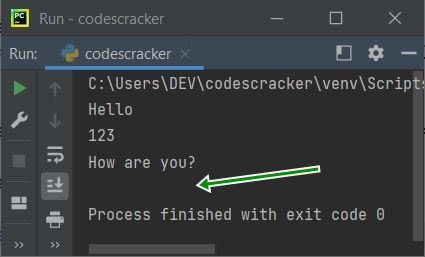But in place of end="\n", if you use end="" in above program, then here is the output you'll see: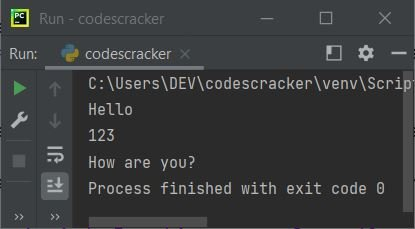Python Online Test

« Previous Function Next Function »